Courses

# NEET Previous Year Questions (2014-20): Magnetism and Matter Class 12 Notes | EduRev

## NEET : NEET Previous Year Questions (2014-20): Magnetism and Matter Class 12 Notes | EduRev

The document NEET Previous Year Questions (2014-20): Magnetism and Matter Class 12 Notes | EduRev is a part of the NEET Course Physics Class 12.
All you need of NEET at this link: NEET

Q.1. An iron rod of susceptibility 599 is subjected to a magnetising field of 1200 A m-1. The permeability of the material of the rod is :      (2020)
0 = 4π×10-7 T mA-1)
A: 2.4π×10-5 T m A-1
B: 2.4π×10-7 T m A-1
C: 2.4π×10-4 T m A-1
D: 8.0×10-5 T m A-1
Ans: C
xm = 599, μ0 = 4π × 10-7
H = 1200 A/m, μ = ?
μ = μ0 (1 + xm)
= 4π × 10-7 (1 + 599)
= 4π × 10-7 × 600
= 24π × 10-5
μ = 2.4π × 10-4 TmA-1

Q.2. At a point A on the earth's surface the angle of dip σ = + 25°. At a point B on the earth's surface the angle of dip, σ = - 25°. We can interpret that :     (2019)
A: A and B are both located in the northern hemisphere.
B: A is located in the southern hemisphere and B is located in the northern hemisphere.
C: A is located in the northern hemisphere and B is located in the southern hemisphere.
D: A and B are both located in the southern hemisphere.
Ans:
C
Solution: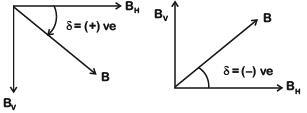point A is located in the Northern hemisphere and point B is located in the southern hemisphere.
basically, angle of dip is the angle made by resultant earth's magnetic field from horizontal.

• angle of dip at equator is considered as zero.
• angle of dip in northern hemisphere is considered as positive.
• angle o dip in southern hemisphere is considered as negative.

here, at point A on the earth's surface,
angle of dip is +25° (i.e., positive) so, point A is located in the Northern hemisphere
and point B on the earth's surface, angle of dip is -25° (i.e., negative) so, point B is located in he southern hemisphere.

Q.3. A carbon resistor (47 + 4.7) kΩ is to be marked with rings of different colours for its identification. The colour code sequence will be :-    (2018)
A: Violet – Yellow – Orange – Silver
B: Yellow – Violet – Orange – Silver
C: Yellow – Green – Violet – Gold
D: Green – Orange – Violet – Gold
Ans:
B
Solution: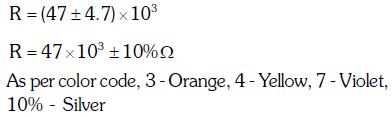Q.4. If θ1 and θ2 be the apparent angles of dip observed in two vertical planes at right angles to each other, then the true angle of dip θ is given by :-     (2017)
A: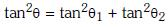B: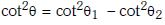C: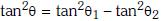D: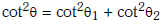Ans: D
Solution: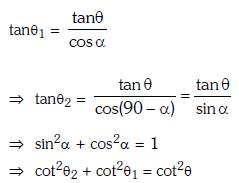Q.5. The magnetic susceptibility negative for    (2016)
A: paramagnetic and ferromagnetic materials
B: diamagnetic material only
C: paramagnetic material only
D: ferromagnetic material only

Ans: B
Solution:
μr = 1 + x
so, μr is negative if and only if  x is negative and x is negative only for diamagnetic materials.

Offer running on EduRev: Apply code STAYHOME200 to get INR 200 off on our premium plan EduRev Infinity!

## Physics Class 12

132 videos|307 docs|150 tests

,

,

,

,

,

,

,

,

,

,

,

,

,

,

,

,

,

,

,

,

,

;